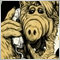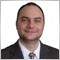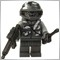# How to calculate tick value by price?[Deleted]

I have to evaluate max profit value by one order's Lots, Ask (Bid) and TakeProfit value. (TakeProfit value = TP x Point)

If this order is a take profit order, the order closed price will equal to Ask + TP x Point (Bid - TP x Point).

I found a formula: profit = Lots x MarketInfo(Symbol(), MODE_TICKVALUE) x TP

But the tick value is not a constant, I wanna evaluate the tick value before sending this order,

so I need to calculate tick value by TP price.2327

Goldbach:

I have to evaluate max profit value by one order's Lots, Ask (Bid) and TakeProfit value. (TakeProfit value = TP x Point)

If this order is a take profit order, the order closed price will equal to Ask + TP x Point (Bid - TP x Point).

I found a formula: profit = Lots x MarketInfo(Symbol(), MODE_TICKVALUE) x TP

But the tick value is not a constant, I wanna evaluate the tick value before sending this order,

so I need to calculate tick value by TP price.

You are asking to know the future...

U can only know current tick value, but obviously at the moment of opening the order u cannot know what the tick value will be when it closes, since that's in the future.[Deleted]
gordon wrote >>

You are asking to know the future...

U can only know current tick value, but obviously at the moment of opening the order u cannot know what the tick value will be when it closes, since that's in the future.

By running EA with following test code:

Comment("TICKVALUE = " + MarketInfo(Symbol(), MODE_TICKVALUE) + ", 1 / Bid = " + (1 / Bid));

The output:

TICKVALUE = 1.11881853, 1 / Bid = 0.01118944
TICKVALUE = 1.11869337, 1 / Bid = 0.01115063
TICKVALUE = 1.11894372, 1 / Bid = 0.01115698

so I guess that if the TICKVALUE is associated with BID value?2327

Goldbach:

By running EA with following test code:

Comment("TICKVALUE = " + MarketInfo(Symbol(), MODE_TICKVALUE) + ", 1 / Bid = " + (1 / Bid));

The output:

TICKVALUE = 1.11881853, 1 / Bid = 0.01118944
TICKVALUE = 1.11869337, 1 / Bid = 0.01115063
TICKVALUE = 1.11894372, 1 / Bid = 0.01115698

so I guess that if the TICKVALUE is associated with BID value?

Tick value is the value of one tick of the quote currency (accessible via MODE_TICKSIZE), in the account deposit currency. It's the method the broker uses to 'translate' your profit on trading any pair into the currency in your own account, so it is sort of like an 'exchange rate'.[Deleted]

I use Tickvalue (together with Ticksize) in any of my EAs where I need, for example, to build a risk or lotsize formula which is flexible enough to function across any combination of account currency and chart pair.

CB2327

gordon:

You are asking to know the future...

U can only know current tick value, but obviously at the moment of opening the order u cannot know what the tick value will be when it closes, since that's in the future.

One clarification regarding my comment - since Tick value acts as an 'exchange rate', if the quoted currency is the SAME as the deposit currency, Tick value will be fixed (and in those cases u can use it in your calculations). But in other cases it will be variable and only it's current value can be known (or if u save it to file, the values in the past).[Deleted]
gordon wrote >>

One clarification regarding my comment - since Tick value acts as an 'exchange rate', if the quoted currency is the SAME as the deposit currency, Tick value will be fixed (and in those cases u can use it in your calculations). But in other cases it will be variable and only it's current value can be known (or if u save it to file, the values in the past).

Thanks,

I found a solution: What's the difference between MODE_TICKSIZE & MODE_POINT?435

One application is when you want to place Stop Loss calculated as an amount of money. Let's say SL at \$135.

Another application is when you want the stop to be percentage from your balance. Example SL at 10% of current balance.

So we have to convert the money to pips. The main problem is to find that "exchange rate".

I'm using this code but it's radder complicated:

```///
/// The Account Percent Stop limits the loss to a
/// predefined percent of the account balance.
/// Returns the number of pips for the StopLoss.
double AccountPercentStopPips(string symbol, double percent, double lots)
{
double balance  = AccountBalance();
double exchrate = AccountExchangeRate(symbol);
double lotsize  = MarketInfo(symbol, MODE_LOTSIZE);
double point    = MarketInfo(symbol, MODE_POINT);

double stopLossPips = percent * balance * exchrate / (lots * lotsize * point) - spread;

return (stopLossPips);
}

/// Gets the Account Exchange Rate
/// AccountExchangeRate = AccountCurrency / QuotedCurrency
/// It serves to convert the profit of a deal in account currency.
/// This code has to be improved!
double AccountExchangeRate(string symbol)
{
if (StringLen(symbol) != 6)
return (1);

string accCurrency    = AccountCurrency();
string baseCurrency   = StringSubstr(symbol, 0, 3);
string quotedCurrency = StringSubstr(symbol, 3, 3);

if (accCurrency == quotedCurrency)
return (1);
else if (accCurrency == baseCurrency)
return (MarketInfo(symbol, MODE_BID));
else
{
string pair = StringConcatenate(accCurrency, quotedCurrency);
double rate = MarketInfo(pair, MODE_BID);

LastError = GetLastError();
if (LastError == 4106)
{
pair = StringConcatenate(quotedCurrency, accCurrency);
rate = MarketInfo(pair, MODE_BID);
LastError = GetLastError();
if (LastError == 0)
rate = 1 / rate;
else
rate = 1;
}
else if (LastError != 0)
rate = 1;

return (rate);
}

return (1);
}
```
This code doesn't work with no forex symbols. Any ideas for improvement?2634

I'm doing something similar in my bot.

```//+------------------------------------------------------------------+
//|Limits the order loss to a predefined percent of the account balance.
//|MarketInfo(Symbol(),MODE_TICKVALUE) will reflect the leverage currently in use.
//|and is the value of one tick (per MODE_LOTSIZE) of the counter currency (per MODE_TICKSIZE) in the account deposit currency
//|It's the method the broker uses to 'translate' your profit on trading any pair into the account currency, its like an 'exchange rate'.
//|MODE_TICKVALUE is calculated using Bid values. If calculated using Ask, it will have minor discrepancy compared to calling MODE_TICKVALUE - Spread.
//|This is because we buy from market at bid, sell back to market at ask i.e. always pay spread
//|This is because we sell to market at ask, buy back from market at bid i.e. always pay spread
//|Point is the smallest possible price movement. MODE_TICKSIZE can be greater than Point.
//|MarketInfo(pair, MODE_TICKVALUE) / MarketInfo(pair, MODE_TICKSIZE)
//+------------------------------------------------------------------+
double AccountPercentStopPoints(string symbol,double percent,double lots) export
{
double AccountRisk=(percent/100)*AccountBalance();
double lotsize=MarketInfo(symbol,MODE_LOTSIZE);
double point=MarketInfo(Symbol(),MODE_POINT);
double exchrate=1/(MarketInfo(symbol,MODE_TICKVALUE));

if(Digits==3)
point=point/100;

double stopLossPoints=NormalizeDouble(((AccountRisk*exchrate)/(lots*lotsize*point)),0);

//Minimal permissible StopLoss value in points.
stopLossPoints=-1;

return (stopLossPoints);
}```23488

`double exchrate=1/(MarketInfo(symbol,MODE_TICKVALUE));`
Do not use tickvalue by itself.
1. You place the stop where it needs to be - where the reason for the trade is no longer valid. E.g. trading a support bounce the stop goes below the support.
2. Account Balance * percent = RISK = (OrderOpenPrice - OrderStopLoss)*DIR * OrderLots * DeltaPerlot (Note OOP-OSL includes the SPREAD)
3. Do NOT use TickValue by itself - DeltaPerlot
4. You must normalize lots properly and check against min and max.
5. You must also check FreeMargin to avoid stop out33

gordon:

Tick value is the value of one tick of the quote currency (accessible via MODE_TICKSIZE), in the account deposit currency. It's the method the broker uses to 'translate' your profit on trading any pair into the currency in your own account, so it is sort of like an 'exchange rate'.

@gordon: My broker has TickValue for Forex pairs in the account deposit currency, but for stocks, ETFs, Energies, Commodities, and Metals it is in the quote currency.  This had me confused for some time until I spotted what was happening.

Is my broker correct, or have they set this up wrong (i.e. should they be using account currency for TickValue in all cases)?

Also, in MT4 'Trade' window ...

1) Is the 'Profit' field stated in the quote currency of the item being traded when the open order is stocks, ETFs, Energies, Commodities, and Metals?

2) Does it use the TickValue to calculate the open Profit, and if so, then would the incorrect TickValue (i.e. not stated in account currency) mean that the calculated Profit/Loss is incorrect?

Thank you.

UPDATE: I just checked one of my trades and the Profit/Loss is being calculated correctly in GBP (for closed trades), so looks like for non-Forex the TickValue is in the quote currency. In order to avoid confusion I would suggest that responses to queries should not assume everyone trades Forex exclusively, as beginners like myself end up making coding errors due to incorrect assumptions from reading forum posts.  Please correct me if I am wrong :=).